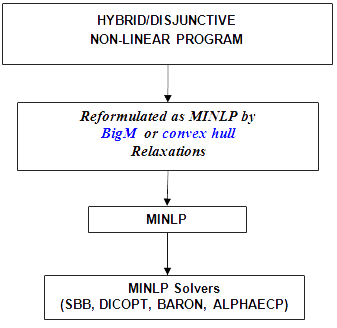# Non-Linear Solvers

The following shows how the solution for non-linear hybrid/disjunctive models is driven:The disjunctions defined in the model are transformed into mixed integer formulations by using one of the relaxations proposed: BigM or convex hull for non-linear problems. The complete set of disjunctions can be transformed by one of those relaxations, or you can choose a different one for each disjunction in the model. Then the problem is converted into a Mixed Integer Non-Linear Program which is later solved by a MINLP solver such that: SBB, DICOPT, BARON, AlphaECP, etc.
Logic Based Outer Approximation algorithm (Turkay and Grossmann, 1996a) does not work anymore for this LogMIP version. A new system is developed, it will be ready for a next release.
The default relaxation is the convex hull. You can change it by introducing in the LogMIP section the following sentence:

DEFAULT Big-M

By means of this sentence disjunctions are relaxed using the Big-M relaxation. Since LogMIP 2.0 belongs to the EMP environment, to solve the problem you must write in the GAMS input file the following two sentences:

OPTION EMP=LOGMIP;
SOLVE modelname USING EMP [MINIMIZING | MAXIMIZING] variablename